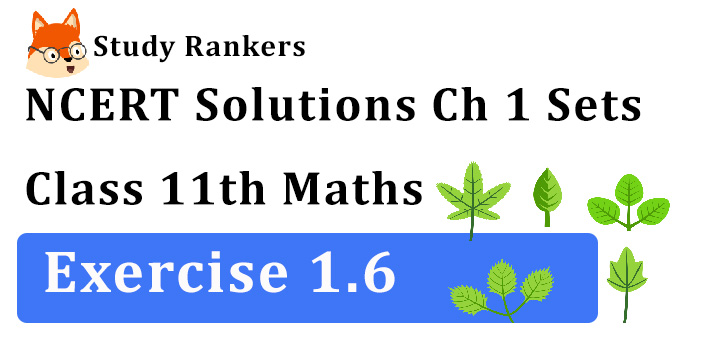## NCERT Solutions for Class 11 Maths Chapter 1 Sets Exercise 1.6

Chapter 1 Sets Exercise 1.6 Class 11 Maths NCERT Solutions that will be helpful completing your homework. NCERT Solutions for Class 11 Maths are as per CBSE curriculum that will help you in scoring better marks in the exams. Studyrankers subject matter experts have prepared accurate and detailed solutions which are updated according to the latest marking scheme.1. If X and Y are two sets such that n (X) = 17, n (Y) = 23 and n (X ∪ Y) = 38, find n (X ∩ Y).

We know that
n (X ∪ Y) = n (X) + n(Y) – n (X ∩ Y)
⇒ 38 = 17 + 23 – n (X ∩ Y).
⇒ 38 = 17 + 23 – n (X ∩ Y)
⇒  n (X ∩ Y) = 40 – 38 = 2.

2. If X and Y are two sets such that X ∪ Y has 18 elements, X has 8 elements and Y has 15 elements; how many elements does X∩Y have?

Here n(X) = 8 and n(Y) = 15
and n (X ∪ Y) = 18
∴ n(X ∪ Y) = n(X) + n(Y) – n(X ∩ Y)
⇒ 18 = 8 + 15 – n(X ∩ Y)
⇒ n(X ∩ Y) = 23 – 18 = 5.

3. In a group of 400 people, 250 can speak Hindi and 200 can speak English. How many people can speak both Hindi and English?

Let H = Set of people who can speak Hindi and E = Set of people who can speak English.
Then, n(H) = 250 n(E) = 200
and n(H ∪ E) = 400
Now, n(H ∪ E) = n (H) + n(E) – n(H ∩ E)
⇒ 400 = 250 + 200 – n (H ∩ E)
⇒ n(H∩ E) = 450 – 400 = 50.
Hence, 50 people can speak both Hindi and English.

4. If S and T are two sets such that S has 21 elements, T has 32 elements, and S ∩ T has 11 elements, how many elements does S ∪ T have?

Here, n (S) = 21 and n(T) = 32
and n(S ∩ T) = 11
Now, n(S ∪ T) = n (S) + n(T) – n(S ∩ T)
= 21 + 32 – 11 = 53 – 11 = 42.

5. If X and Y are two sets such that X has 40 elements, X ∪ Y has 60 elements and X ∩ Y has 10 elements, how many elements does Y have?

Here, n(X) = 40
n(X ∪ Y) = 60
n(X ∩ Y) = 10
Now, n(X ∪ Y) = n(X) + n(Y) – n(X ∩ Y)
⇒ 60 = 40 + n (Y) – 10
⇒ n(Y) = 60 – 40 + 10 = 20 + 10 = 30.

6. In a group of 70 people, 37 like coffee, 52 like tea and each person likes at least one of the two drinks. How many people like both coffee and tea?

Let C = Set of people who like coffee and T
= Set of people who like tea.
Then, n (C ∪ T) = 70, n(C) = 37.
n(T) = 52, n (C ∩ T) =?
Now, we know that
n (C ∪ T) = n(C) + n(T) – n(C ∩ T)
⇒ 70 = 37 + 52 – n(C ∩ T)
⇒ n(C ∩ T) = 89 – 70 = 19
Hence, 19 people like both coffee and tea.

7. In a group of 65 people, 40 like cricket, 10 like both cricket and tennis. How many like tennis only and not cricket? How many like tennis?

Let C = the set of people who like cricket and
T = the set of people who like tennis.
Then, n(C ∪ T) = 65, n(C) = 40
n(C ∩ T) = 10
We know that
n(C ∪ T) = n(C) + n(T) – n(C ∩ T)
⇒ 65 = 40 + n(T) – 10
⇒ n(T) = 65 – 30 = 35
Number of people who like only tennis
= n (T) – n (C ∩ T)
= 35 – 10 = 25
Hence, number of people who like tennis is 35 and number of people who like tennis only is 25.

8. In a committee, 50 people speak French, 20 speak Spanish and 10 speak both Spanish and French. How many speak at least one of these two languages?